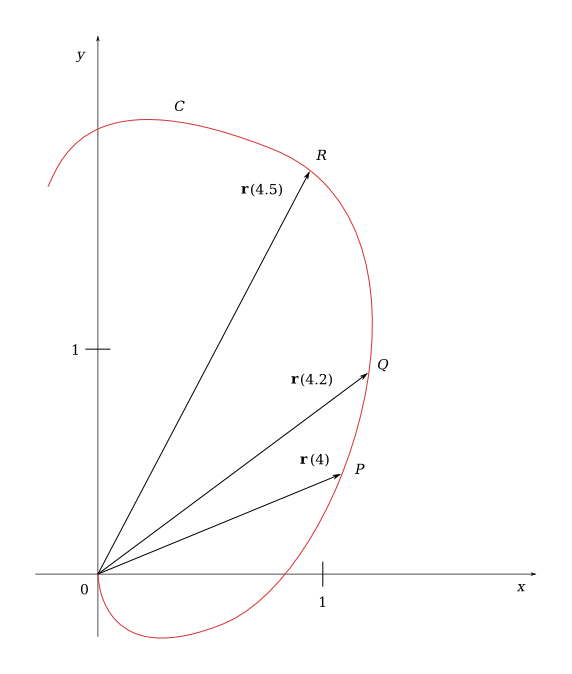Derivatives And Integrals Of Vector Functions

(requires JavaScript)

1. The figure shows a curve $C$ given by a vector function $\mathbf{r}\left(t\right)$.

1. Draw the vectors $\mathbf{r}\left(4.5\right)-\mathbf{r}\left(4\right)$, $\mathbf{r}\left(4.2\right)-\mathbf{r}\left(4\right)$, $\frac{\mathbf{r}\left(4.5\right)-\mathbf{r}\left(4\right)}{0.5}$, and $\frac{\mathbf{r}\left(4.2\right)-\mathbf{r}\left(4\right)}{0.2}$.
2. Write expressions for $\mathbf{r}\prime \left(4\right)$ and the unit tangent vector $\mathbf{T}\left(4\right)$. Draw the vector $\mathbf{T}\left(4\right)$.2. Make a large sketch of the curve described by the vector function $\mathbf{r}\left(t\right)=⟨{t}^{2},t⟩,\phantom{\rule{0.5em}{0ex}}0\le t\le 2$, and draw the vectors $\mathbf{r}\left(1\right)$, $\mathbf{r}\left(1.1\right)$, and $\mathbf{r}\left(1.1\right)-\mathbf{r}\left(1\right)$. Moreover, draw the vector $\mathbf{r}\prime \left(1\right)$ starting at $\left(1,1\right)$ and compare it with the vector

$\frac{\mathbf{r}\left(1.1\right)-\mathbf{r}\left(1\right)}{0.1}$

Explain why these vectors are similar.

3. Let $\mathbf{r}\left(t\right)=\left(1+\mathrm{cos}\left(t\right)\right)\mathbf{i}+\left(2+\mathrm{sin}\left(t\right)\right)\mathbf{j}$ and $t=\frac{\pi }{6}$, sketch the plane curve with the given vector equation, find $\mathbf{r}\prime \left(t\right)$, and sketch the position vector $\mathbf{r}\left(t\right)$ and the tangent vector $\mathbf{r}\prime \left(t\right)$ for the given value of $t$.
4. Find the derivative of the vector function

$\mathbf{r}\left(t\right)={e}^{{t}^{2}}\mathbf{i}-\mathbf{j}+\mathrm{ln}\left(1+3t\right)\mathbf{k}$.

$\mathbf{r}\prime \left(t\right)=2t{e}^{{t}^{2}}\mathbf{i}+\frac{3}{1+3t}\mathbf{k}$
5. Find the unit tangent vector $\mathbf{T}\left(t\right)$ at the point where $t=0$ for the curve given by the equation

$\mathbf{r}\left(t\right)=\mathrm{cos}\left(t\right)\mathbf{i}+3t\mathbf{j}+2\mathrm{sin}\left(2t\right)\mathbf{k}$.

$\frac{3}{5}\mathbf{j}+\frac{4}{5}\mathbf{k}$
6. Find parametric equations for the tangent line to the curve

$x=\mathrm{ln}\left(t\right),\phantom{\rule{0.5em}{0ex}}y=2\sqrt{t},\phantom{\rule{0.5em}{0ex}}z={t}^{2}$

at the point $\left(0,2,1\right)$.

$x=t$, $y=2+t$, $z=1+2t$
7. At what point do the curves

${\mathbf{r}}_{1}\left(t\right)=⟨t,1-t,3+{t}^{2}⟩$

and

${\mathbf{r}}_{2}\left(s\right)=⟨3-s,s-2,{s}^{2}⟩$

intersect? Find the angle of intersection to the nearest degree.

$⟨1,0,4⟩$, the angle is approx. $54.74°$
8. Evaluate the integral

${\int }_{0}^{\pi /2}\left(3{\mathrm{sin}}^{2}\left(t\right)\mathrm{cos}\left(t\right)\mathbf{i}+3\mathrm{sin}\left(t\right){\mathrm{cos}}^{2}\left(t\right)\mathbf{j}+2\mathrm{sin}\left(t\right)\mathrm{cos}\left(t\right)\mathbf{k}\right)\phantom{\rule{0.2em}{0ex}}dt$

$\mathbf{i}+\mathbf{j}+\mathbf{k}$
9. Find $\mathbf{r}\left(t\right)$ if $\mathbf{r}\prime \left(t\right)=t\mathbf{i}+{e}^{t}\mathbf{j}+t{e}^{t}\mathbf{k}$ and $\mathbf{r}\left(0\right)=\mathbf{i}+\mathbf{j}+\mathbf{k}$.
$\mathbf{r}\left(t\right)=⟨\frac{{t}^{2}}{2}+1,{e}^{t},t{e}^{t}-{e}^{t}+2⟩$